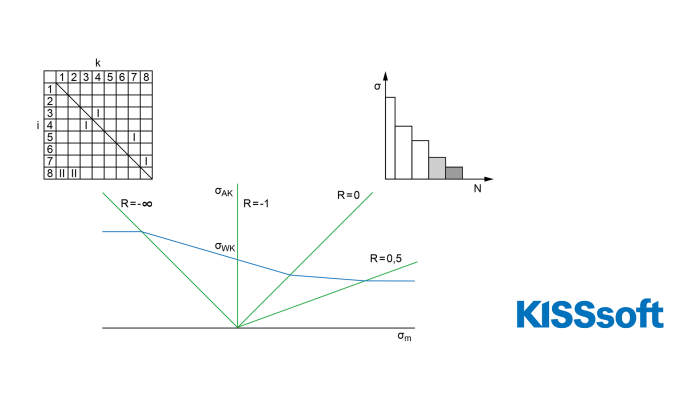# FKM shaft rating with rainflow matrix

Apr 27, 2023

In the KISSsoft shaft calculation, the proof of fatigue strength can be performed by generating an equivalent stress verification according to the FKM Guideline.

This verification is equal to the amplitude verification which has been performed up to now. With the equivalent stress verification, different stress ratios can be taken into account for each load case (for example, a Rainflow matrix). The load cases are defined in a load spectrum, which can be entered directly via a table or imported from a text file.

The influence of different stress ratios can now be considered on the load side of the verification by converting the load spectrum to mean stress Sm = 0 and the stress ratio R = -1. For this purpose, an amplitude factor KAK is calculated for each load case in the load spectrum. Overload case 2, i.e. constant stress ratio, is assumed.

As a result, the strength calculation for a shaft can now be performed according to the FKM Guideline, taking into account the influence of a Rainflow matrix load spectrum.